• 正态随机变量Pseudorandom_number_generators 线性同余生成器 (LCG) 是一种算法，可生成使用不连续分段线性方程计算伪随机数序列。 该方法代表了最古老和最著名伪随机数生成器算法之一。 参考： ...matlab
• 随机变量生成算法——产生离散型随机变量样本值的方法 算法介绍 设离散型随机变量X具有分布律 现在来产生X随机数： 先产生伪随机数u，令 由于 所以X具有给定分布律。 下面将引用浙大版概统（第四版）中例题...
随机变量生成算法——产生离散型随机变量样本值的方法
算法介绍
设离散型随机变量X具有分布律现在来产生X的随机数：
先产生伪随机数u，令由于所以X具有给定的分布律。
下面将引用浙大版概统（第四版）中的例题为例1、计算过程2、C++代码实现
#include<bits/stdc++.h>
int main()
{
float x,y,z,u;
x=1;
y=2;
z=3;
for(int i=0;i<10000;i++)
{
x[i+1]=fmod(171*x[i],30269);
y[i+1]=fmod(170*y[i],30307);
z[i+1]=fmod(172*z[i],30323);
u[i+1]=fmod(x[i+1]/30269+y[i+1]/30307+z[i+1]/30323,1);
}
FILE *fp;
fp=fopen("/*输入自定义路径*/","w");
for(int i=1;i<=10000;i++)
{
if(u[i]<0.2)
fprintf(fp,"1 ");
else if(u[i]<0.35)
fprintf(fp,"2 ");
else if(u[i]<0.6)
fprintf(fp,"3 ");
else
fprintf(fp,"4 ");
}//模拟算法部分
fclose(fp);
return 0;
}

3、使用matlab画直方图
u=[…(从C++程序生成文件中导入数据)]
histogram(u)

4、生成直方图预览展开全文matlab c++
• 方便获得任意参数的广义高斯分布随机变量，基于伽玛分布推导出广义高斯分布随机变量的生成算法，利用变换法和舍选抽样法，给出伽玛分布随机变量的生成方法．该算法计算简单，通过调整分布参数值，可产生具有任何形状...
• 计算统计的基本工具就是要从指定的概率分布中生成随机变量，最基本最重要的是均匀分布的伪随机数生成器，其他概率分布的随机数生成方法大都依赖于均匀分布随机数生成器。 在R语言中，均匀分布的随机数的生成为： run...
统计计算系列文章目录

第一话 统计计算之随机变量生成方法

提示：写完文章后，目录可以自动生成，如何生成可参考右边的帮助文档
统计计算之随机变量生成方法统计计算系列文章目录1 引言2 随机数生成方法-逆变换方法(Inverse Transform Method)2.1  laplace分布随机数2.2 Pareto(a, b) 分布随机数3 转换方法4 混合方法5 生成wishart分布随机数6 随机过程齐次泊松过程（Poisson Processes）非齐次泊松过程（Poisson Processes）更新过程（Renewal Processes）
（本篇博客是自己根据导师的统计计算课程的课件自行整理可得，如有错误，请联系我，此笔记仅用于学习，如需转载，请注明来源，谢谢！）
1 引言
计算统计的基本工具就是要从指定的概率分布中生成随机变量，最基本最重要的是均匀分布的伪随机数生成器，其他概率分布的随机数生成方法大都依赖于均匀分布随机数生成器。
在R语言中，均匀分布的随机数的生成为：
runif(n) #generate a vector of size n in [0,1]
runif(n,a,b) #generate n Uniform(a, b) numbers
matrix(runif(n*m),n,m) #generate n by m matrix

另外，一个抽样函数sample()用于从一个有限总体中有放回或者无放回地抽样。
sample(0:1, size = 10, replace = TRUE) # replace = TRUE 表示有放回抽样
sample(1:100, size = 6, replace = FALSE) # replace = FALSE 表示有放回抽样
x=sample(1:3,size=100,replace=TRUE,prob=c(.2,.3,.5)) #sample from a multinomial dist.

2 随机数生成方法-逆变换方法(Inverse Transform Method)
逆变换方法主要使用以下定理：

定理1：如果X是一个连续的随机变量，其CDF(累积分布函数)为$F_{X}(x)$，则$U = F_{X}(X) \sim U(0,1)$。

关于定理1的证明，在此不再详细赘述，有兴趣可参见相关统计计算书籍。
根据定理1，得到一般连续型随机变量$X$的生成步骤：

step1: 推导逆函数$F_{X}^{-1}(u)$；
step2: 生成一个来自均匀分布$U(0,1)$的随机数$u$
step3: 通过步骤1的逆函数产生$X$的随机数$x=F_{X}^{-1}(u)$

这个方法是生成随机数的最基本的方法，通常很容易应用在分布函数的逆$F_X^{-1}$很容易计算的前提下。
2.1  laplace分布随机数
标准的Laplace分布的密度函数为$f(x)=\frac{1}{2} e^{-|x|}, x \in \mathbb{R}$。使用逆变换方法从这个分布中生成样本量为1000的随机样本。并使用本章演示的方法来检查和比较生成的样本和目标分布。
根据标准的Laplace分布的密度函数通过求积分可以得知其分布函数为：
$L a(x ; 0,1)=\left\{\begin{array}{ll} \frac{1}{2} e^{x}, & x \leq 0 \\ 1-\frac{1}{2} e^{-x}, & x>0 \end{array}\right.$
根据以上分布函数，求逆函数如下：
$F_X^{-1}=\left\{\begin{array}{ll}\log (2 u) & 0
根据以上逆函数，可以采用逆变换方法生成随机数,通过密度直方图对生成的随机数进行检验：：
set.seed(20201021)
u= runif(1000) # generate 1000 random numbers form U(0,1)
x <- ifelse(u<=0.5,log(2*u),log(1/(2*(1-u))))
mi =floor(min(x))
ma = ceiling(max(x))
hist(x,probability = TRUE,ylim = c(0,0.6),xlim = c(mi,ma),main = bquote(f(x)==0.5*e^{-abs(x)}),
breaks = seq(mi,ma,by = 0.5))
data.frame(mean(x),sd(x))
y <- seq(-10,10,0.2)
lines(y,0.5*exp(-abs(y)),lwd = 2) #density curve f(x)2.2 Pareto(a, b) 分布随机数
Pareto(a, b) 分布的cdf如下：
$F(x)=1-\left(\frac{b}{x}\right)^{a}, \quad x \geq b>0, a>0$
推导概率逆变换函数$F^{-1}(U)$，并且使用逆变换方法来模拟生成Pareto(2, 2)的随机数。用Pareto(2，2)密度叠加的样本密度直方图进行比较。
通过计算Pareto(a, b) 分布F(X)的逆$F^{-1}(U)$得到：
$F^{-1}(U) = \frac{b}{(1-u)^{1/a}}$
假设$a = 2,b = 2$,则产生Pareto随机数如下：
n = 1000;a = 2;b =2
set.seed(20201021)
u = runif(n)
x = b/(1-u)^(1/a) # the pareto random numbers
# hist(x,probability = TRUE,breaks = seq(0, ceiling(max(x)), 1))
ma = ceiling(max(x))
hist(x,probability = TRUE,breaks = seq(0,ma,1))
y = seq(0,ma,1)
lines(y,a*b/y^(a+1),lwd = 2)3 转换方法
例：
由对数正态分布的性质可知，假设$X = e^{Y},Y \sim N\left(\mu, \sigma^{2}\right),\log{X} \sim N\left(\mu, \sigma^{2}\right)$,因此要想产生对数正态分布的随机数，必须先产生正态分布随机数。在此假设$\mu = 0,\sigma = 1$.
rlognormal = function(n,mu,sd){
y = rnorm(n,mean = mu,sd = sd)
x = exp(y)
return(x)
}
n = 1000
x = rlognormal(n,0,1)
ma = ceiling(max(x))
hist(x,probability = TRUE,xlim = c(0,ma),ylim = c(0,.8),breaks =  seq(0, ma,0.5))
# curve(dlnorm(x),add = TRUE,lwd = 2)
d = seq(from = min(x),to = max(x), length = n)
lines(d,dlnorm(d,meanlog = 0,sdlog = 1),lwd = 2)4 混合方法
例：模拟连续的Exponential-Gamma mixture，假设比例参数$\Lambda$ 服从 $\operatorname{Gamma}(r, \beta)$分布，$Y$ 服从 $\operatorname{Exp}(\Lambda)$ 分布。$(Y \mid \Lambda=\lambda) \sim f_{Y}(y \mid \lambda)=\lambda e^{-\lambda y}$，给定$r = 4,\beta = 2$,从这个混合分布中产生1000个观测值。
set.seed(20201021)
r = 4; beta = 2
n = 1000
lambda = rgamma(n,r,beta)
x = rexp(n,lambda)
hist(x,probability = TRUE)
#check : Exponential-Gamma mixture is the Pareto distribution,so use the pdf of Pareto distribution
y = seq(min(x),max(x),length = n)
lines(y,64/(2+y)^5)
#library(actuar)
#lines(y,dpareto(y,shape = r,scale = 2))5 生成wishart分布随机数
写一个基于Bartlett 分解的函数用于生成$W_d(\Sigma,df)$(Wishart)分布的随机样本（$n>d+1>=1$）
r.Wishart = function(n,df,Sigma){
# n : the sample size
# df: the freedom of Wishart distribution
#Sigma: the parameter of Wishart distribution
d = nrow(Sigma)
x = array(0,dim = c(d,d,n))
for (i in 1:n) {
T = x[,,i]
Tij = rnorm(d*(d-1)/2,0,1)
T[which(lower.tri(T))] = Tij # upper triangular elements in place of Tij
len = 1:d
df2 = df - len + 1
Tii = sqrt(rchisq(d,df2))
diag(T) = Tii # the elements of diagonal in place of Tii
A = T %*% t(T)
L = t(chol(Sigma)) # evaluate the lower triangular matrix
x[,,i] = L %*% A %*% t(L)
}
return(x)
}

调用r.Wishart()函数的示例。
Sigma = matrix(c(5,1,1,3),2,2) # the covirance parameters for Wishart distribution
df = 50  # the freedom parameters for Wishart distribution
n = 1000 # sample size
set.seed(20201021)
x = r.Wishart(n,df,Sigma)

检查生成的Wishart分布的正确性主要采用Wishart分布的均值性质：$W \sim W_p(df,\Sigma),则E(W) = df*\Sigma$
EWhat = apply(x,1:2,mean)  # Empirical result
EW = df*Sigma   # real result
list(EWhat = EWhat,EW = EW)6 随机过程
计数过程记录在时间t之前发生的事件或到达的次数。下面介绍两个基本概念：独立增量和平稳增量

独立增量： 如果在不相交的时间间隔内到达的次数(或事件的次数)是独立的，则计数过程具有独立的增量。
平稳增量：如果间隔中发生的事件次数仅取决于间隔的长度，则计数过程具有平稳增量。

计数过程的一个例子是泊松过程。
为了通过模拟研究计数过程，我们可以生成一个在一定时间内记录事件的过程的实现(relization)。 连续到达的时间集记录了结果并确定任意时间$t$时的状态$X(t)$。 在模拟中，到达的时间的顺序必须是有限的。 模拟计数过程的一种方法是选择足够长的时间间隔，并在此间隔中生成到达时间或到达间隔时间。
齐次泊松过程（Poisson Processes）
速率为$\lambda$的齐次Poisson过程${N（t）,t≥0}$是一个具有独立增量的计数过程，使得N（0）= 0且
到达时间T1，T2，…是连续到达之间的时间。到达的间隔时间是比率为λ的独立同分布的指数分布，其取自上式和指数分布的无记忆特性。
模拟一个泊松过程的一种方法是生成到达的间隔时间。 那么，第n次到达的时间为总和Sn = T1 + … + Tn（直到第n次到达的等待时间）。 到达的时间序列$\{T_n\}_{n =1}^{∞}$或到达时间序列$\{S_n\}_{n =1}^{∞}$是该过程的实现。 但是，实现是一个无限的序列，而不是单个数字。 在仿真中，到达时间$\{T_n\}_{n =1}^{N}$或到达时间$\{S_n\}_{n =1}^{N}$的有限序列是间隔$[0，S_N]$上该过程的仿真实现。
模拟Poisson过程的另一种方法是利用以下事实：给定N（t）= n，（无序）到达时间的条件分布与Uniform（0，t）中大小为n的随机样本的条件分布是一样的。
给定时间t的过程状态等价于在[0，t]区间上的到达次数，即min（k：Sk> t）-1。 即，N（t）＝ n-1，其中Sn是超过t的最小到达时间。这个方法使用for 循环来执行算法生成到达的间隔时间，算法的速度较慢，可以采用向量化编程。
下面的代码阐述了一种简单的方法来模拟带有速率为$\lambda$的泊松过程。
假设我们需要得到$N(3)$,也就是在区间[0,3]上的到达次数。生成独立同分布的指数时间$T_i$（速率为$\lambda$）并且找到索引$n$，也即是累积求和$S_n = T_1 +...+T_n$首次超过3。那么在[0,3]区间上到达的次数就是$n-1$，并且这个次数的平均值为$E[N(3)]=3\lambda$
lambda = 2 # the value of rate lambda
t0 = 3 # set the end time
Tn = rexp(100,lambda) # generate the iid exponential Ti with rate lambda
Sn = cumsum(Tn) # arrival time
n = min(which(Sn>t0))
x = n -1 # the number of arrivals in [0,3]
round(Sn[1:n],4) #arrival time for per arrival

产生泊松过程的到达时间的另一种方法是基于以下事实：给定间隔（0，t）中的到达次数，无序的到达时间的条件分布是(0,t)上的均匀分布。假设给定(0,t)上的到达次数为n,到达时间$S_1,S_2,S_3,...,S_n$是联合分布作为一个样本量为n的有序的随机样本来自于uniform(0,t).
应用到达时间的条件分布，可以通过首先从Poisson（λt）分布生成随机观测值n，来模拟区间（0，t）上的Poisson（λ）过程，然后生成n个来自于均匀（0，t）的随机样本，并对随机样本进行排序以获得到达时间。
lambda= 2
t0 = 3
upper = 100
pp = numeric(10000){
N = rpois(1, lambda * upper)
Un = runif(N, 0, upper) # unordered arrival times
Sn = sort(Un)    # arrival times
n = min(which(Sn>t0)) # arrivals +1 in [0,t0]
pp[i] = n-1   # arrivals in [0,t0]
}

pp = replicate(10000,expr = {
N = rpois(1, lambda * upper)
Un = runif(N, 0, upper) # unordered arrival times
Sn = sort(Un)    # arrival times
n = min(which(Sn>t0)) # arrivals +1 in [0,t0]
n-1   # arrivals in [0,t0]
})

非齐次泊松过程（Poisson Processes）
更新过程（Renewal Processes）


展开全文• 其中一种可以用来产生随机变量的方法是逆变换法。在本文中，我将向您展示如何使用Python中的逆变换方法生成随机变量(包括离散和连续的情况)。 概念 给定随机变量U，其中U在（0,1）中均匀分布。 假设我们要生成随机...
目标
在仿真理论中，生成随机变量是最重要的“构建块”之一，而这些随机变量大多是由均匀分布的随机变量生成的。其中一种可以用来产生随机变量的方法是逆变换法。在本文中，我将向您展示如何使用Python中的逆变换方法生成随机变量(包括离散和连续的情况)。
概念
给定随机变量U，其中U在（0,1）中均匀分布。 假设我们要生成随机变量X，其中累积分布函数（CDF）为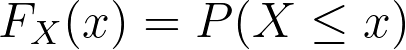逆变换方法的思想是通过如下使用其逆CDF从任何概率分布中生成一个随机数。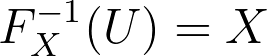对于离散随机变量，步骤略有不同。假设我们想生成一个离散随机变量X的值，它具有一个概率质量函数(PMF)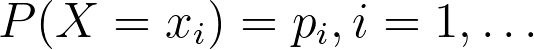为了生成X的值，需要生成一个随机变量U，U在（0,1）中均匀分布，并且定义通过以上步骤，我们可以按如下方法创建逆变换方法的算法。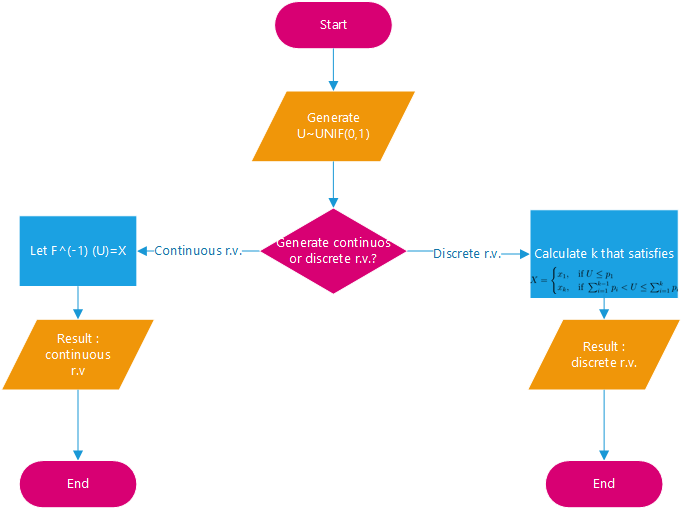连续随机数代码实现
首先，我们实现此方法以生成连续随机变量。 假设我们要模拟一个随机变量X，该变量遵循均值λ（即X〜EXP（λ））的指数分布。 我们知道指数分布的概率分布函数（PDF）是CDF如下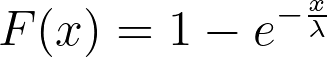然后，我们可以使用以下的方法写出逆CDF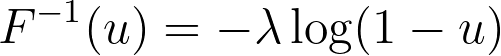在Python中，我们可以通过如下编写这些代码行来简单地实现它。
### Generate exponential distributed random variables given the mean
### and number of random variables
def exponential_inverse_trans(n=1,mean=1):
U=uniform.rvs(size=n)
X=-mean*np.log(1-U)
actual=expon.rvs(size=n,scale=mean)

plt.figure(figsize=(12,9))
plt.hist(X, bins=50, alpha=0.5, label="Generated r.v.")
plt.hist(actual, bins=50, alpha=0.5, label="Actual r.v.")
plt.title("Generated vs Actual %i Exponential Random Variables" %n)
plt.legend()
plt.show()
return X

我们可以通过运行以下示例来尝试上面的代码。 请注意，由于我们要生成随机变量，因此结果可能会有所不同。
cont_example1=exponential_inverse_trans(n=100,mean=4)
cont_example2=exponential_inverse_trans(n=500,mean=4)
cont_example3=exponential_inverse_trans(n=1000,mean=4)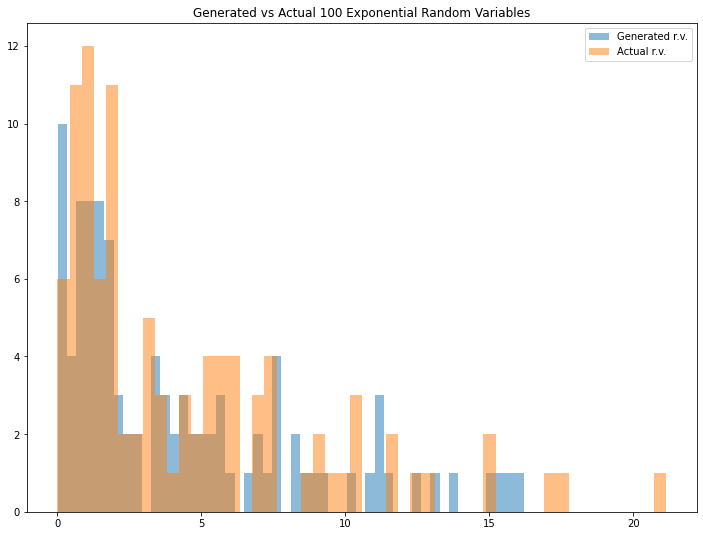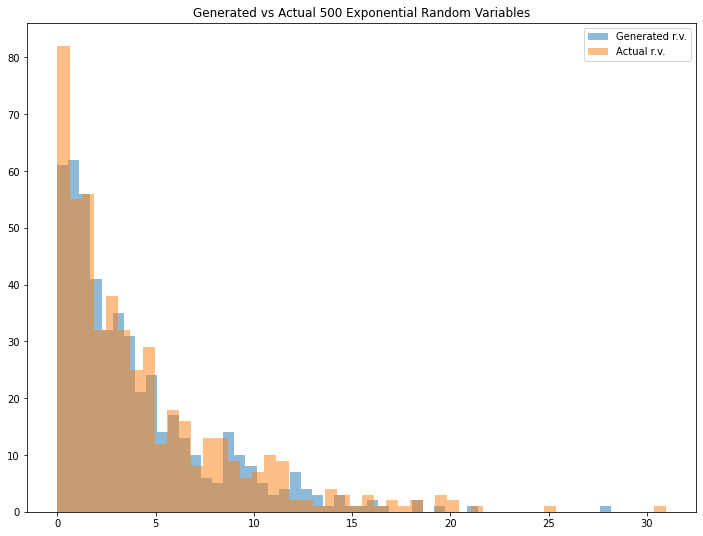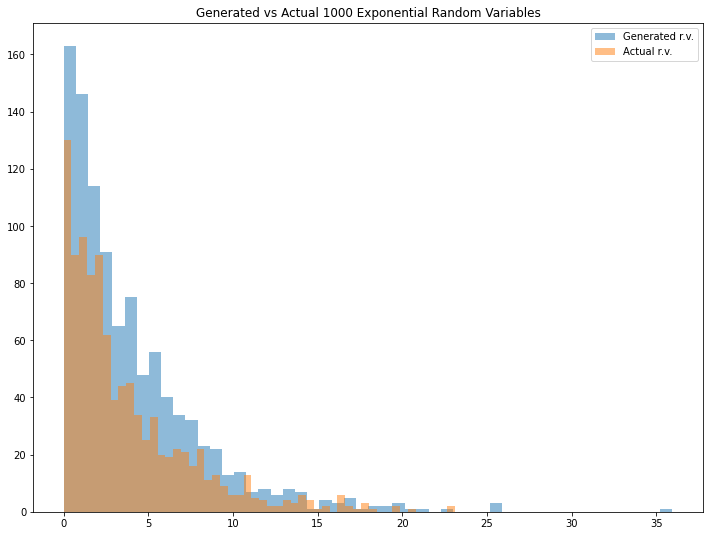看起来很有趣。 如果将其与实际变量进行比较，我们可以看到生成的随机变量具有非常相似的结果。 可以调整均值（请注意，我为expon.rvs（）函数定义的均值是指数分布中的比例参数）和/或 生成的随机变量的数量，以查看不同的结果。
离散随机数实现代码
对于离散随机变量情况，假设我们要模拟遵循以下分布的离散随机变量情况X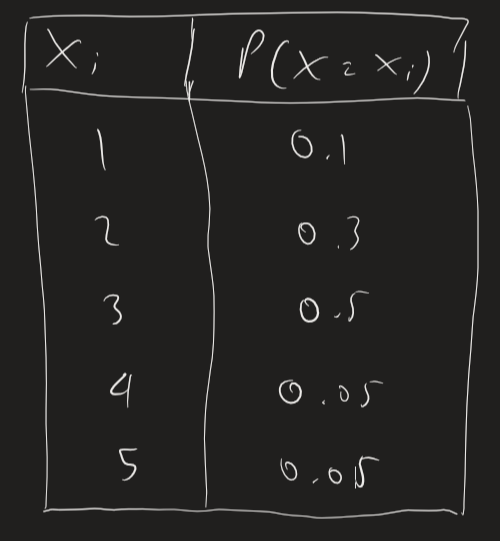首先，我们编写函数以使用这些代码行为一个样本生成离散随机变量。
### Generate arbitary discrete distributed random variables given
### the probability vector
def discrete_inverse_trans(prob_vec):
U=uniform.rvs(size=1)
if U<=prob_vec:
return 1
else:
for i in range(1,len(prob_vec)+1):
if sum(prob_vec[0:i])<U and sum(prob_vec[0:i+1])>U:
return i+1

然后，我们创建一个函数以使用这些代码行生成许多随机变量样本。
def discrete_samples(prob_vec,n=1):
sample=[]
for i in range(0,n):
sample.append(discrete_inverse_trans(prob_vec))
return np.array(sample)

最后，我们创建一个函数来模拟结果，并通过这些代码行将其与实际结果进行比较。
def discrete_simulate(prob_vec,numbers,n=1):
sample_disc=discrete_samples(prob_vec,n)
unique, counts=np.unique(sample_disc,return_counts=True)

fig=plt.figure()
ax=fig.add_axes([0,0,1,1])
prob=counts/n
ax.bar(numbers,prob)
ax.set_title("Simulation of Generating %i Discrete Random Variables" %n)
plt.show()

data={'X':unique,'Number of samples':counts,'Empirical Probability':prob,'Actual Probability':prob_vec}
df=pd.DataFrame(data=data)
return df

我们可以在下面运行一些示例以查看结果。 同样，请注意，由于我们要生成随机变量，因此结果可能会有所不同。
prob_vec=np.array([0.1,0.3,0.5,0.05,0.05])
numbers=np.array([1,2,3,4,5])

dis_example1=discrete_simulate(prob_vec, numbers, n=100)
dis_example2=discrete_simulate(prob_vec, numbers, n=500)
dis_example3=discrete_simulate(prob_vec, numbers, n=1000)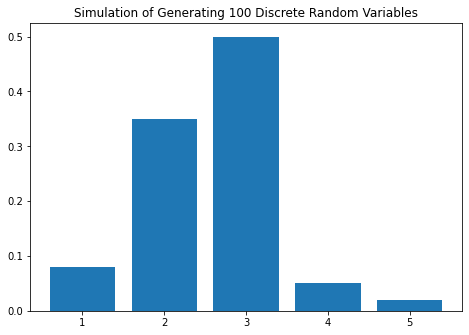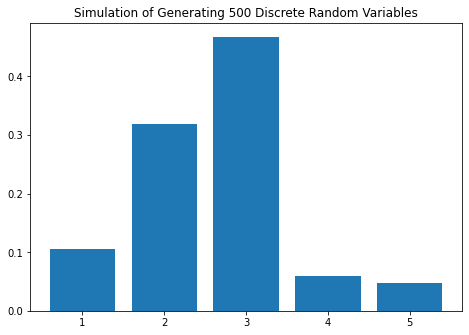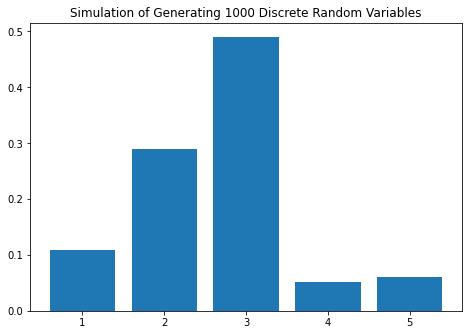In: dis_example1
Out:
X  Number of samples  Empirical Probability  Actual Probability
0  1                  8                   0.08                0.10
1  2                 35                   0.35                0.30
2  3                 50                   0.50                0.50
3  4                  5                   0.05                0.05
4  5                  2                   0.02                0.05In: dis_example2
Out:
X  Number of samples  Empirical Probability  Actual Probability
0  1                 53                  0.106                0.10
1  2                159                  0.318                0.30
2  3                234                  0.468                0.50
3  4                 30                  0.060                0.05
4  5                 24                  0.048                0.05In: dis_example3
Out:
X  Number of samples  Empirical Probability  Actual Probability
0  1                108                  0.108                0.10
1  2                290                  0.290                0.30
2  3                491                  0.491                0.50
3  4                 51                  0.051                0.05
4  5                 60                  0.060                0.05

结果很有趣！ 我们可以看到，随着我们增加随机变量样本的数量，经验概率越来越接近实际概率。 尝试使用不同数量的样本和/或不同的分布进行实验，以查看不同的结果。
总结
这种逆变换方法是统计中非常重要的工具，尤其是在仿真理论中，在给定随机变量均匀分布在（0,1）中的情况下，我们想生成随机变量。 研究案例本身非常广泛，您可以使用在生成经验累积分布函数，预测分析中使用到的这种方法。
作者:Raden Aurelius Andhika Viadinugroho
deephub翻译组


展开全文python 数据挖掘 机器学习
• 该文件包含使用两种方法生成随机变量的各种函数：逆方法和接受拒绝方法。 包括来自以下分布的随机变量： 连续均匀分布； 指数分布； 标准正态分布； 泊松分布； 广义帕累托分布； 伯努利分布； 二项分布; 贝塔分布；...matlab
• 本公众号MyEncyclopedia定期发布AI，算法，工程类深度和前沿文章。学习本文最佳姿势为点击文末在看，发送本文链接到桌面版浏览器，打开文末阅读原文，敲入代码运行。上期 从零构建统...


本公众号MyEncyclopedia定期发布AI，算法，工程类深度和前沿文章。学习本文的最佳姿势为点击文末在看，发送本文链接到桌面版浏览器，打开文末阅读原文，敲入代码运行。上期 从零构建统计随机变量生成器之离散基础篇，我们从零出发构建了基于伯努利的基础离散分布，这一期我们来详细介绍广泛使用的 Inverse Transform Method（逆变换采样方法）。逆变换采样方法Inverse Transform Method 是最基础常见的方法，可用于离散分布和连续分布。常见的分布一般都能通过此方法生成，只需要随机变量CDF的解析表达式。假设随机变量 ，其CDF为 ，则 Inverse Transform Method 仅有两步通过生成 [0, 1] 之间的均匀随机数代入  即产生满足分布的实例离散例子我们先举一个离散分布来直观感受一下其工作机制。有如下PMF的离散类别分布，范围在 [1, 5]。转换成CDF为画出对应的CDF图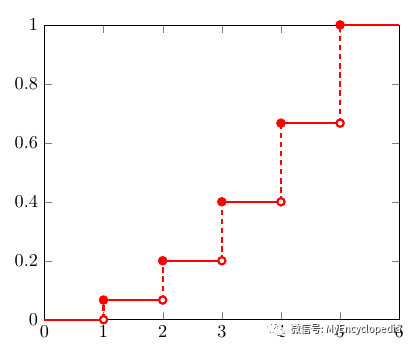那么Inverse Transformation Method 的第一步，随机生成 0-1 之间的数 u，可以直观的认为是在 y 轴上生成一个随机的点 u。注意到5段竖虚线对应了5个离散的取值，它们的长度和为1，并且每一段长度代表了每个值的权重。因此，通过在 y 轴上的均匀采样可以生成给定PMF的 x 的分布。离散分布的逆变换采样方法用数学公式可以表述为：找到第一个 x，其CDF的范围包括了 u，即扩展到连续分布有了离散类别分布的直观感受，扩展到连续分布也就不难理解了。类似于微积分中将连续空间做离散切分，再通过极限的方法，连续光滑函数在 y 轴上可以切分成长度为  的线段，那么生成的 x 值就是其近似值。随着 ，最终  即为满足要求的分布。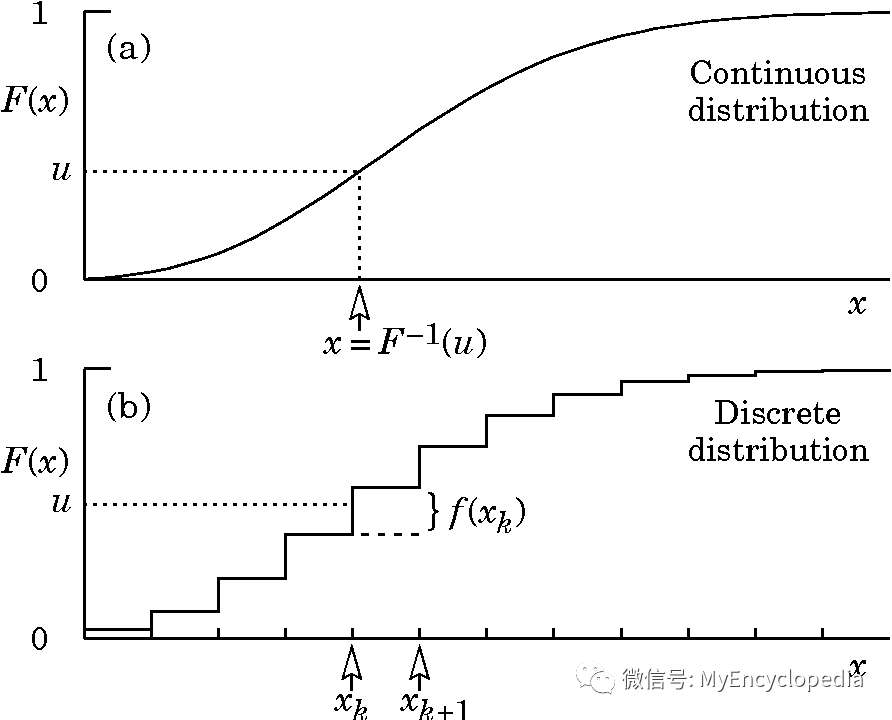指数分布（连续）以最为常见的指数分布为例，我们来看看具体的步骤。我们知道指数分布的PDF如下PDF 图为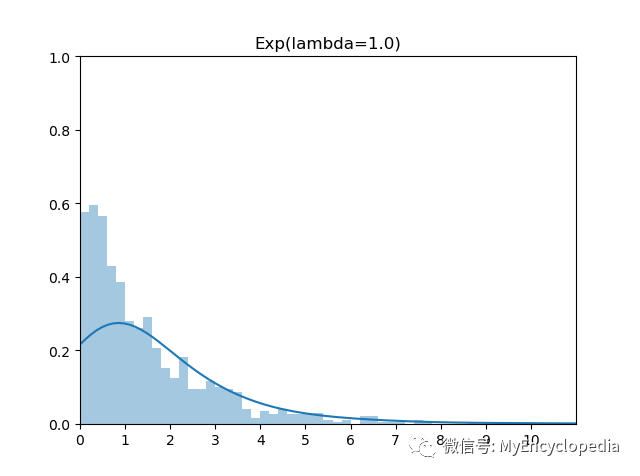计算CDF为CDF 图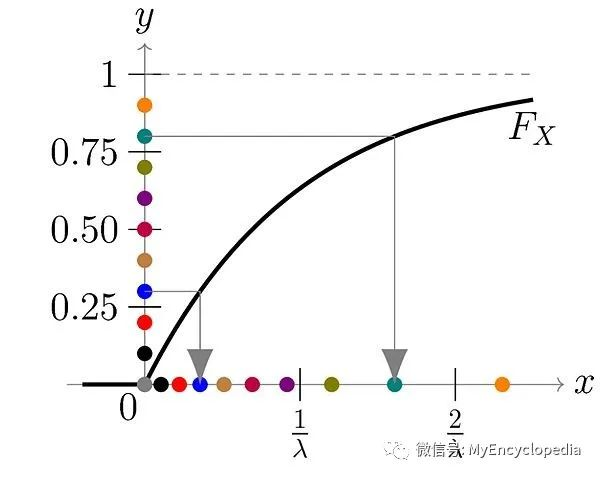可以求得逆函数为由于 1-u 在 [0, 1] 范围上的随机数等价于 u，因此，x 的生成公式等价于实现代码对应代码很简单import random
from math import log2 as ln

def exp_gen(lambbda: float) -> float:
u = random.random()
return -ln(u) / lambbda
Github 代码地址：https://github.com/MyEncyclopedia/stats_simulation/blob/main/distrib_sim/coutinous_exp_inv.py持续模拟动画类别分布（离散）我们再来看基于类别分布 Inverse Transformation Method的其他离散分布例子。在从零构建统计随机变量生成器之离散基础篇中，我们已经介绍了类别分布（Categorical Distribution）的逆变换采样算法，同时还介绍了通过模拟 Bernoulli 实验来生成二项，几何，超几何分布的方法。在这一篇中，我们通过逆变换采样算法再来生成这些分布。先回顾一下类别分布的逆变换采样实现。给定如下的类别分布，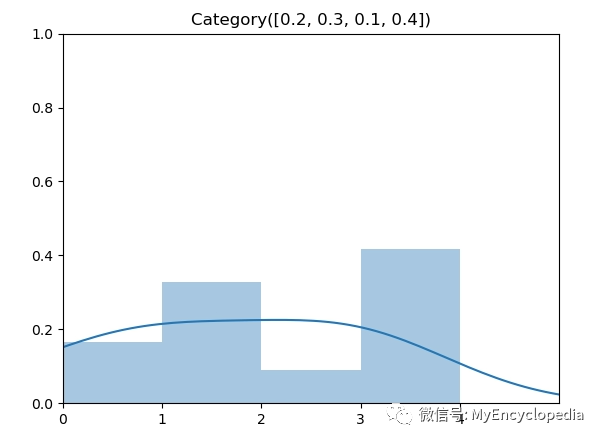实现代码类别分布的逆变换采样实现需要找到第一个大于 u 的元素的索引序号，在我们的实现中，将  转换成累计概率  后，由于  数组是非递减的，因此我们可以用二分法代替线性查找，将时间复杂度降到 。下面的实现中直接调用 python bisect 函数即可。import bisect
import random
from typing import List

def categorical(probs: List[float]) -> int:
assert abs(sum(probs) - 1.0) < 0.001
cum = probs.copy()

for i in range(1, len(cum)):
cum[i] = cum[i-1] + probs[i]

u = random.random()
return bisect.bisect(cum, u)
Github 代码地址：https://github.com/MyEncyclopedia/stats_simulation/blob/main/distrib_sim/discrete_categorical.py二项分布（离散）二项分布（Binomial Distribution）有两个参数 n 和 p，表示伯努利实验做n次后成功的次数。图中为 n=6，p=0.5的二项分布。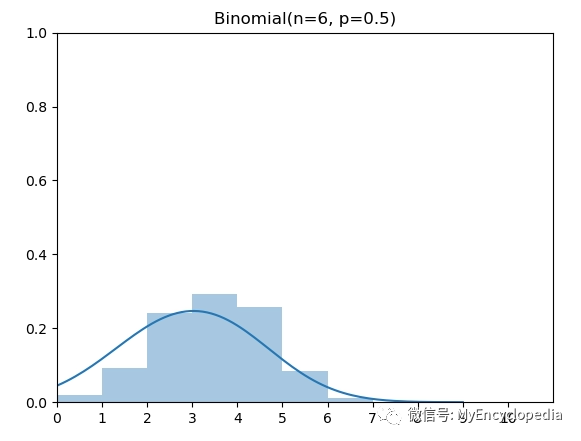概率质量函数（PMF）实现代码根据上面的PMF定义，我们将 [0, 6] 上的PMF计算出来，然后调用类别分布的逆变换采样实现即可：from scipy.special import comb

from discrete_categorical import categorical
from math import pow

def binomial(n: int, p: float) -> int:
pmf = [comb(n, k, exact=True) * pow(p, k) * pow(1-p, n-k) for k in range(0, n + 1)]
return categorical(pmf)
Github 代码地址：https://github.com/MyEncyclopedia/stats_simulation/blob/main/distrib_sim/discrete_binomial_inv.py持续模拟动画超几何分布（离散）同样的，超几何分布（HyperGeometric Distribution）也可以如法炮制。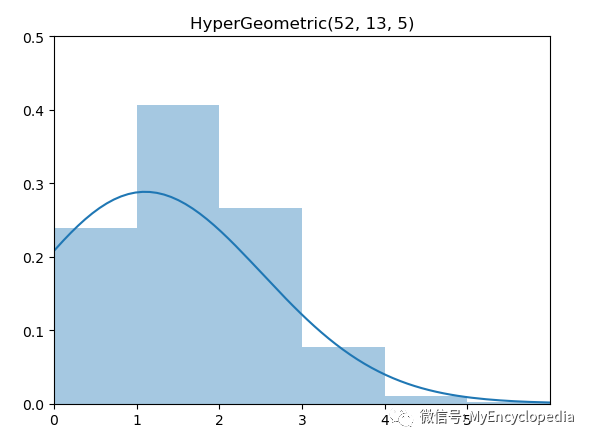概率质量函数（PMF）实现代码from scipy.special import comb

from discrete_categorical import categorical

def hypergeometric(N: int, K_succ_num: int, n_trial_num: int) -> int:
pmf = [comb(K_succ_num, k, exact=True) * comb(N - K_succ_num, n_trial_num - k, exact=True) / comb(N, n_trial_num, exact=True)
for k in range(max(0, n_trial_num - (N - K_succ_num)), min(K_succ_num, n_trial_num) + 1)]
return categorical(pmf)
Github 代码地址：https://github.com/MyEncyclopedia/stats_simulation/blob/main/distrib_sim/discrete_hypergeometric_inv.py持续模拟动画几何分布（离散）几何分布（Geometric Distribution）和上面的二项分布以及超几何分布不同的是，它的 support 是所有非负整数，因此，我们无法穷举计算所有 x 的概率。但是，我们可以通过将CDF 推出 Inverse CDF的解析表达式来直接实现。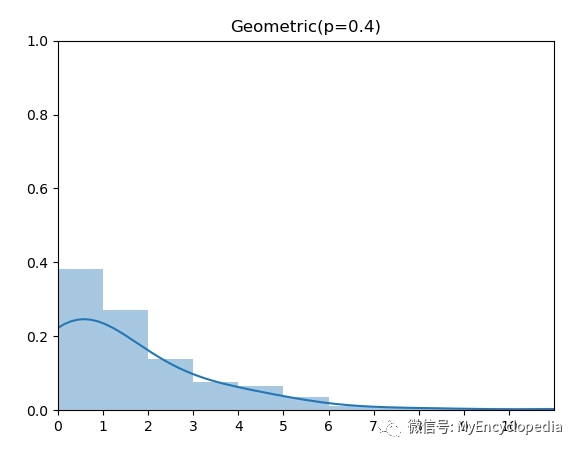概率质量函数（PMF）CDFInverse CDF反函数求得为实现代码import random
from math import floor
from math import log2 as ln

def geometric(p: float) -> int:
u = random.random()
return floor(ln(u) / ln(1-p))
Github 代码地址：https://github.com/MyEncyclopedia/stats_simulation/blob/main/distrib_sim/discrete_geometric_inv.py持续模拟动画标准正态分布一般，标准正态分布用Box-Muller 方法来生成，这个后续将做详细介绍。这里我们用 Schmeiser 提出的基于Inverse Transformation Method的近似方法来生成：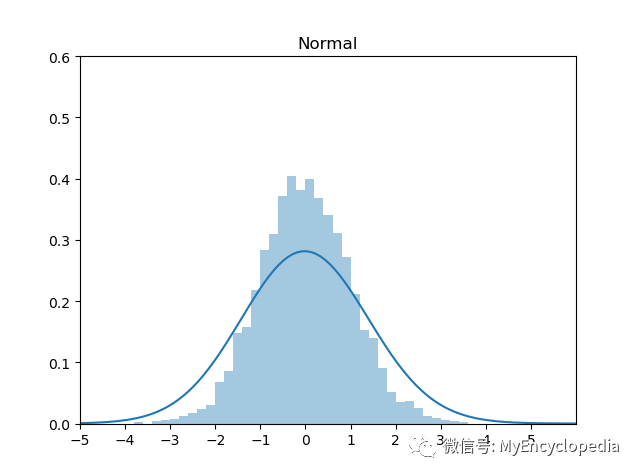实现代码import random

def normal():
import math
u = random.random()
return (math.pow(u, 0.135) - math.pow(1-u, 0.135)) / 0.1975
Github 代码地址：https://github.com/MyEncyclopedia/stats_simulation/blob/main/distrib_sim/coutinous_normal_apprx.py持续模拟动画著作权归作者所有。商业转载请联系作者获得授权，非商业转载请注明出处。

展开全文算法 python 机器学习 深度学习 random
• 函数变换法是关于随机变量的函数的抽样法。通过随机变量间的关系式可以导出其分布函数之间的关系式，故可利用常用分布的随机数生成某个确定分布的随机数。 以指数分布随机数生成为例，由于U(0，1)是常见分布函数，...
• 许多计算统计方法需要从已知概率分布中生成随机变量，这也是用于统计推断（statistical inference)的蒙特卡罗方法的核心。...生成均匀随机变量的相关方法在[Gentle, 1998]中有详尽的讨论。 生成均匀分布随机变...
• 可以从一个随机变量（例如X）生成随机数。当可能结果总数有限时，P（X = x）大于零，范围在零到一之间。在这种情况下，随机变量X被认为是离散。当可能结果总数为无限时，P（X = x）恰好为零。在这种情况下，...
• 输入： (N,M) = 要生成的随机变量数组大小b = 比例参数 > 0 c = 形状参数 > 0 概率密度函数 (pdf) p(x) = (x/b)^(c-1) * exp(-x/b) / (b * gamma(c)) 其中 gamma(c) 是 gamma 函数（ ...matlab
• RanLip 使用接受/拒绝方法生成随机向量，该方法基于使用“帽子”函数从上方逼近概率密度... 它对多达五个变量表现出良好性能，并为用户提供了一个黑匣子用于一大类分布非均匀随机变量生成器，特别是多峰分布。matlab
• 这是离散事件仿真与建模的实验报告，里面有源程序跟代码，主要是掌握随机数、随机变量的生成方法，用到反变换法、拒绝法的机理。
• 您可以使用此软件包中软件从(1) 相关多元二元随机变量（multivariate Bernoulli） (2) 相关多元泊松随机变量(3) 将随机变量与任意边际统计相关联。 应用包括人工神经数据建模和生成。 实现包括二分高斯分布...matlab
• 针对故障树分析方法性能评价研究中存在测试基准规模和多样性问题，基于故障树样本随机生成的思想，确定了故障树6个主要结构特征，并依据这些结构特征给出了自顶向下地生成算法，包括随机树骨架生成算法和随机重复...
• 以下几种方式可以随机生成字符串。当然，还要许多方法都能完成文章标题目的，这里仅列举出来部分。 1.使用shellrandom变量 [root@virmach ~]# echo $RANDOM 1908 2.使用openssl [root@virm... • 基于二维分布讨论了Sklar定理，介绍了由Sklar定理直接生成Copula函数的方法以及生成给定边际分布联合分布函数的方法 • 本文实例讲述了Python编程生成随机用户名及密码的方法。分享给大家供大家参考，具体如下： 方案一： import random global userName,userPassword #为了便于使用，定义为全局变量 userName = '' userPassword = '' ... • 服从正态分布随机的生成生成单变量正态分布随机数Box-Muller 算法Accept/Reject 方法Marsaglia-Bray 算法附录Box-Muller 算法的简要证明 生成单变量正态分布随机数 Box-Muller 算法 Box-Muller算法是利用两个i.i.d...统计学 python • <?... /** array unique_rand( int$min, int $max, int$num )* 生成一定数量不重复随机数* $min 和$...//随机生成不重复N个数function unique_rand($min,$max, $num) { //初始化变量为0$count = 0; 　...
• 了解随机数的生成方法，掌握二变量多种信息量的计算方法，并用C/C++程序实现 二、实验内容： 用C/C++语言（其他语言也可以）实现： （1）根据如下的联合概率密度 分别计算X与Y的熵、联合熵、条件熵：H（X）、H（Y）...
• 如果要生成某一范围内的随机整数情况，下面是本人常用几种方法。 【方法一】使用内部系统变量RANDOM来生成 示例1: 生成一个1-1024范围内的随机整数. 【方法二】使用python -c参数，借助于
• 一种生成正态随机数的方法 生成步骤 第一步： 产生两个（0，1）上独立均匀分布变量u1和u2 第二步：考虑如下两个变量 X1=(−2loge⁡(u1))1/2cos⁡(2πu2)" role="presentation" ...matlab
• 竟然有小伙伴喜欢在编写代码时使用随机字符当作类名、方法名、变量名，例如这一篇博客里代码：使用 Resharper 特性 - 林德熙。既然随机，那也随机得像一些啊！于是我改进了标识符的随机算法，使得生成的标识符更像...
• TDIST 计算独立对称（零均值）随机变量 (RV) 线性组合分布（PDF、CDF 或 QF - 分位数），例如 Y = sum_i \lambda_i X_i，其中 X_i 具有特定分布： - 0 < df < Inf 学生 t 分布， -正态分布N（0,1）， - ...matlab...